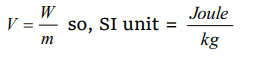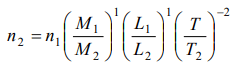## Units and Measurement Questions and Answers Part-9

1. Which of the following is not the unit of energy
a) Calorie
b) Joule
c) Electron volt
d) Watt

Explanation: Watt is a unit of power

2. Which of the following is not a unit of time
a) Leap year
b) Micro second
c) Lunar month
d) Light year

Explanation: 1 light year = 9.46 * 1015 meter

3. The S.I. unit of gravitational potential is
a) J
b) $J-kg^{-1}$
c) $J-kg$
d) $J-kg^{-2}$

Explanation:4. Which one of the following is not a unit of young's modulus
a) $Nm^{-1}$
b) $Nm^{-2}$
c) Dyne $cm^{-2}$
d) Mega Pascal

Explanation: $Nm^{-1}$

5. In C.G.S. system the magnitutde of the force is 100 dynes. In another system where the fundamental physical quantities are kilogram, metre and minute, the magnitude of the force is
a) 0.036
b) 0.36
c) 3.6
d) 36

Explanation:6. The unit of L/R is (where L = inductance and R = resistance)
a) sec
b) $sec^{-1}$
c) Volt
d) Ampere

Explanation: [L/R] is a time constant so its unit is Second

7. Which is different from others by units
a) Phase difference
b) Mechanical equivalent
c) Loudness of sound
d) Poisson's ratio

Explanation: Poission ratio is a unitless quantity

8. Length cannot be measured by
a) Fermi
b) Debye
c) Micron
d) Light year

Explanation: Length cannot be measured by Debye

9. The value of Planck's constant is
a) $6.63\times 10^{-34} J -sec$
b) $6.63\times 10^{34} J\diagup sec$
c) $6.63\times 10^{34} kg\diagup sec$
d) $6.63\times 10^{-34} kg- m^{2}$

Explanation: $6.63\times 10^{-34} J -sec$
10. A physical quantity is measured and its value is found to be nu where $n=$ numerical value and $u=$ unit. Then which of the following relations is true
a) $n\propto u^{2}$
b) $n\propto u$
c) $n\propto\sqrt{u}$
d) $n\propto\frac{1}{u}$